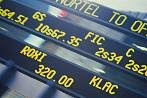## Tuesday, 23 January 2007

### How CFDs WorkLet's take Microsoft as an example and we assume the price of Microsoft stock is \$30.00:

First, we have to decide whether we want to sell (going short) or buy (going long) the stock:

We assume we will go LONG i.e. buy the stock. We also decide to stake \$1.00 on each point of the fluctuation on Microsoft's share price. This means for every Cent that Microsoft share moves, we will gain or lose \$1:00 depending on the direction of the movement.

Now to make it clear, we assume Microsoft share price has gone up by 10 Cents by the end of the day, if we wish we can now take profits, so let's assume we do this:

Therefore, our profit is:

10 x 1 = \$10.00